Courses

# Power System Stability Of Power Systems

## 10 Questions MCQ Test Topicwise Question Bank for Electrical Engineering | Power System Stability Of Power Systems

Description
This mock test of Power System Stability Of Power Systems for Electrical Engineering (EE) helps you for every Electrical Engineering (EE) entrance exam. This contains 10 Multiple Choice Questions for Electrical Engineering (EE) Power System Stability Of Power Systems (mcq) to study with solutions a complete question bank. The solved questions answers in this Power System Stability Of Power Systems quiz give you a good mix of easy questions and tough questions. Electrical Engineering (EE) students definitely take this Power System Stability Of Power Systems exercise for a better result in the exam. You can find other Power System Stability Of Power Systems extra questions, long questions & short questions for Electrical Engineering (EE) on EduRev as well by searching above.
QUESTION: 1

### A 500-MVA synchronous machine has H1 = 4.6 MJ/MVA, and a 1500 MVA machine has H2 = 3.0 MJ/MVA. The two machines operate in parallel in a power station. The equivalent H constant for the two, relative to a 100 MVA base will be

Solution:

We know that,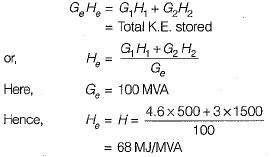QUESTION: 2

Solution:
QUESTION: 3

### Fast acting excitation systems

Solution:

Fast field excitation system is one of the method to improve transient stability of the system.

QUESTION: 4

A shunt reactor in general

Solution:

Shunt reactors are used to reduce the effect of Ferranti effect when a medium or a long line is lightly loaded or is on no-load. It doesn’t affect the steady state power transfer limit.

QUESTION: 5

The angle swings during transients

Solution:
QUESTION: 6

If the inertia constant H = 9.0 MJ/MVA for a 50 MVA generator, the stored energy is

Solution: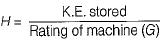or,
K.E. stored = GH = 50 x 9 = 450 MJ

QUESTION: 7

Bundled conductors in a transmission line will

Solution:

Bundled conductors increases self GMD due to which the series inductive reactance is reduced as a result of which power transferred from sending end to the receiving end is increased. Hence, it improves the steady state stability of the system.

QUESTION: 8

A 1500 MVA synchronous generator has an inertia constant of 5.0 MJ/MVA. The inertia constant of the machine relative to a 50 MVA base is

Solution:

Since inertia constant,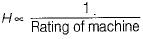∴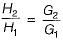or,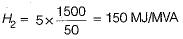QUESTION: 9

If the initial acceleration power of a 100 MVA, 50 Hz synchronous generator having H= 5 MJ/MVA is Xpu, the initial acceleration in elec-deg/s2 and the inertia constant in M J-s/ele-deg respectively will be

Solution:

From swing equation,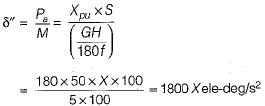Also, inertia constant,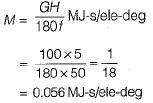QUESTION: 10

The critical clearing angle of a power system is related to

Solution:

tcr and δcr are related to transient stability limit. The fault in a power system must be cleared before δ reaches δcr and before tcr.